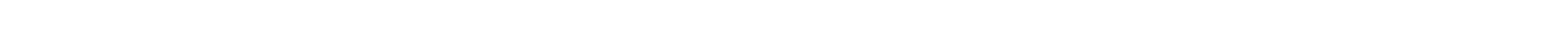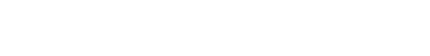# Decision Trees

Decision tree is the building block of random forest algorithm, and is considered to be one of the most popular algorithms in machine learning, which is used for classification purposes.

Visualize it this way- It works like a human brain before any decision is made on the task at hand.

The idea behind using decision tree is to divide the input dataset into smaller dataset based on specific feature value until every target variable fall under one single category. This split is made so as to get the maximum information gain for every step.

Every decision tree begins with a root name, which is the place where the first split is made. An efficient way needs to be determined to make sure that the nodes are defined properly. This is where Gini comes into picture.

Gini is considered to be the most commonly used measurement that helps measure inequality. Inequality here refers to the target class which every subset in a node would belong to. Hence, the Gini value is calculated after every split. Based on how the Gini value/ the inequality value changes after every node, information gain can be defined.

### How is Gini value calculated?

The probability of finding a class for every node split is taken, its sum is squared and this value is subtracted from 1. Hence, the subset is a pure subset, which means it contains just one class inside it. The Gini value would be 0, since probability of finding that specific class is actually 1.

This means the lowermost node or the leaf has been reached. After this, there is no possibility or way to split the node further. Therefore, the decision tree would have been built.

Instead of Gini value, another value can be used to calculate the inequality of classes, and this is known as ‘entropy’. Gini value and Entropy serve the same purpose but vary slightly with respect to the scale.

Depending on which splitting strategy has been chosen, different values of Gini can be obtained for every subset of the data, and this value changes after every node. Information Gain can be defined as the different between Gini value of the parent node and the weighted average of the child nodes of the Gini values.

All possible splits of the data nodes are considered by the decision tree and the one that has the highest information gain is considered.

### Implementing a simple Decision Tree

Let us look at how a simple decision tree can be implemented with the help of a code example:

from sklearn.tree import DecisionTreeClassifier
import pandas as pd
#Matrix of the input dataset is created
data = [[8,8.68,'abc'],[50,41,'dabcog'],[7.9,9,'xyz'],[15,13,'abc'],[8.9,9.8,'xyz']]
#A dataframe is generated
df = pd.DataFrame(data, columns = ['weight','height','label'])
#The predictors are defined
X = df[['weight','height']]
#The target variable is defined and is mapped to 'abc' and 'xyz' y = df['label'].replace({'dog':1, 'cat':0})
#The model is instantiated
tree = DecisionTreeClassifier()
#The model is fit on the data
model = tree.fit(X,y) 

A dataframe was built which was made to fit the model. From the code, a few observations need to be made:

The DecisionTreeClassifier was instantiated without providing any parameters to it. When the input data set is too large, the user has to control the tree from growing and overfitting. This is when the ‘max_depth’ parameter has to be considered, which help specify the number of splits that can be made to the decision tree. The ‘max_features’ parameter can also be set so that the number of predictors can also be maintained and controlled. The criterion can be defined as ‘entropy’ instead of ‘gini’ to change the inequality measure used.

Consider the below code example:

from sklearn.externals.six import StringIO
from sklearn.tree import export_graphviz
import pydotplus
from IPython.display import Image
dot_data = StringIO()
export_graphviz(
model,
out_file = dot_data,
filled=True, rounded=True, proportion=False,
special_characters=True,
feature_names=X.columns,
class_names=["cat", "dog"]
)
graph = pydotplus.graph_from_dot_data(dot_data.getvalue())
Image(graph.create_png()) 

This generates a decision tree that helps differentiate between ‘abc’ and ‘xyz’ values.

• Easy to interpret
• Deal well with noisy and incomplete data
• It can be used to implement classification as well as regression algorithms.

• Sometimes, it can be unstable, i.e a small change in the data can make a big difference in the model
• Sometimes, it tends to overfit with low bias and high variance. It might not perform well on never-before-seen data but may train well.

#### Conclusion

In this post, we understood what decision trees are, their significance, advantages and disadvantages with the help of code examples.#### Vinu

After reading your article, I was amazed. I know that you explain it very well. And I hope that other readers will also experience how I feel after reading your article. Thanks for sharing.#### Johnson M

Good and informative article.#### Vinu

I enjoyed reading your articles. This is truly a great read for me. Keep up the good work!#### Vinu